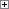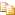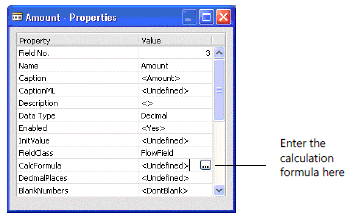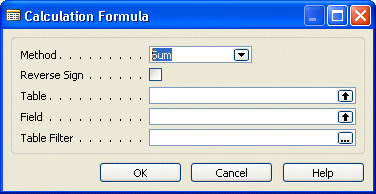The following procedure describes the steps to take to create and revise a calculation formula for a FlowField of a table.

To create, view, and edit a calculation formula

1. On the Tools menu, choose Object Designer. Open the table to which you want to add a calculation formula.

2. Select the field for which you want to create, view, or edit the calculation formula.

3. On the View menu, choose Properties to open the Properties window.

4. Find the CalcFormula property.5. Enter the calculation formula directly or choose the AssistEdit button. When you choose the AssistEdit button, the following dialog box is displayed.6. Choose the drop-down button to select the appropriate calculation method.

7. Select the Reverse Sign option to reverse the sign of the result (only for Sum and Average).

8. Use the up arrows to select the table and field from which to get the information.

9. If necessary, you can add a table filter to specify a limited set of records. Choose the AssistEdit button to the right of the Table Filter field and the Table Filter window opens.10. On each line in this window, you can define a field filter. For each field filter, specify a field, a type, and a value. You can also set the OnlyMaxLimit and the ValueIsFilter options.

The following example illustrates where the information in the Table Filter window comes from. The Balance at Date field in the G/L Account table is a decimal type FlowField. This field is calculated from values in the Amount Column in the G/L Entry table.Some of the fields in the G/L Account table are FlowFilter fields. By entering filter expressions into these fields, the user can affect the calculation of FlowFields field, such as Balance at Date, at run time. The user can enter filter values on a FlowFilter page.If the user enters a date filter expression in the Date Filter field, it is transferred via the table filter and used in the Date column in the G/L Entry table.

You can use the OnlyMaxLimit option to remove the lower limit from a range defined by a filter expression. For example, if the filter expression is defined as a range x..y, setting the OnlyMaxLimit option transforms the expression into ..y.

The ValueIsFilter option determines how the system interprets the contents of the field referred to in the Value column in the Table Filter window. For example, if the field contains the value 1000..2000, setting the ValueIsFilter option will cause this value to be interpreted as a filter rather than as a specific value.